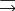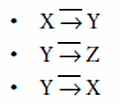Functional, Transitive, and Multivalued Compatibilities | Teradata Vantage - 17.10 - Functional, Transitive, and Multivalued Compatibilities - Advanced SQL Engine - Teradata Database

Product
Release Number
17.10
Release Date
July 2021
Content Type
User Guide
Publication ID
B035-1094-171K
Language
English (United States)

Definitions

Term Definition
Determinant For any relation R, the set of attributes X in the functional dependency X Y is the determinant group for the dependency.
Full functional dependence An attribute set X is fully functionally dependent on another attribute set Y if X is functionally dependent on the whole set of Y, but not on any subset of Y.
Functional dependence For any relation R, attribute X is functionally dependent on attribute Y if for every valid instance, the value of Y determines the value of X.

The symbolic notation for this is Y   X.

Multivalued dependence For any relation R with attribute set (X,Y,Z), the set X multivalue determines the set Y if, for every pair of tuples containing duplicates in X, the instance also contains the pair of tuples obtained by interchanging the Y tuples in the original pair.

The symbolic notation for this is X  Y.

Transitive dependence Transitive dependencies are dependencies that exist among three or more attributes in a relation in such a way that one attribute determines a third by way of an intermediate attribute. For example, consider the relation R(X,Y,Z), where X is the primary key.

Attribute Z is transitively dependent on attribute X if attribute Y satisfies the following dependencies, where the symbolindicates "does not determine":Inference Axioms for Functional Dependencies

The concept of functional dependencies for relations produced a complete set of inference axioms for functional and multivalued dependencies for relations. These axioms are described in the following table:

Axiom Formal Expression
Reflexive rule X  X
Augmentation rule IF X →  Y THEN XZ  Y
Union rule IF X  Y AND X →  Z THEN X →  YZ
Decomposition rule IF X  Y THEN X  Z WHERE Z is a subset of Y
Transitivity rule IF X  Y AND Y  Z THEN X →  Z
Pseudotransitivity rule IF X  Y AND YZ  W THEN XZ  W
For the sake of some examples, assume the attribute set R, S, T, U, V with the following set of dependencies:
• R   S
• TU   R
• T   V
• V   T
• SU   T

The following table lists many of the dependencies among these attributes implied by the inference axioms:

Dependency Supporting Axiom
R  R Reflexive rule
RT  S Augmentation rule
TU  RV
• Augmentation rule
• Union rule
RU  T Pseudotransitivity rule
UV  R Pseudotransitivity rule
TU  S Transitivity rule
SU  V Transitivity rule
VU  R Pseudotransitivity rule
RU  V
• Transitivity rule
• Pseudotransitivity rule
UV  S
• Transitivity rule
• Pseudotransitivity rule

Inference Axioms for Multivalued Dependencies

The following table lists the complete set of multivalued dependencies:

Axiom Formal Expression
Reflexive rule X  X
Augmentation rule IF X ⇒  Y THEN XZ ⇒  Y
Union rule IF X ⇒  Y AND X  Z THEN X ⇒  YZ
Decomposition rule IF X ⇒  Y AND X  Z THEN X ⇒  Y Z AND X ⇒  (Z - Y)
Transitivity rule IF X ⇒  Y AND Y ⇒  Z THEN X ⇒  (Z - Y)
Pseudotransitivity rule IF X ⇒  Y AND YW ⇒  Z THEN XW ⇒  (Z - YW)
Complement rule IF X  Y AND Z R-XY THEN X ⇒  Z
Mixed inference rules IF X  Y THEN X  Y
IF X  Y AND Z ⇒  W

WHERE W is contained in Y AND Y Z is not empty

THEN XW